## Top Ad unit 728 × 90

### Project Goal: To develop a software pipeline to identify the lane boundaries in a video from a front-facing camera on a car. Detect lane lines in a variety of conditions, including changing road surfaces, curved roads, and variable lighting.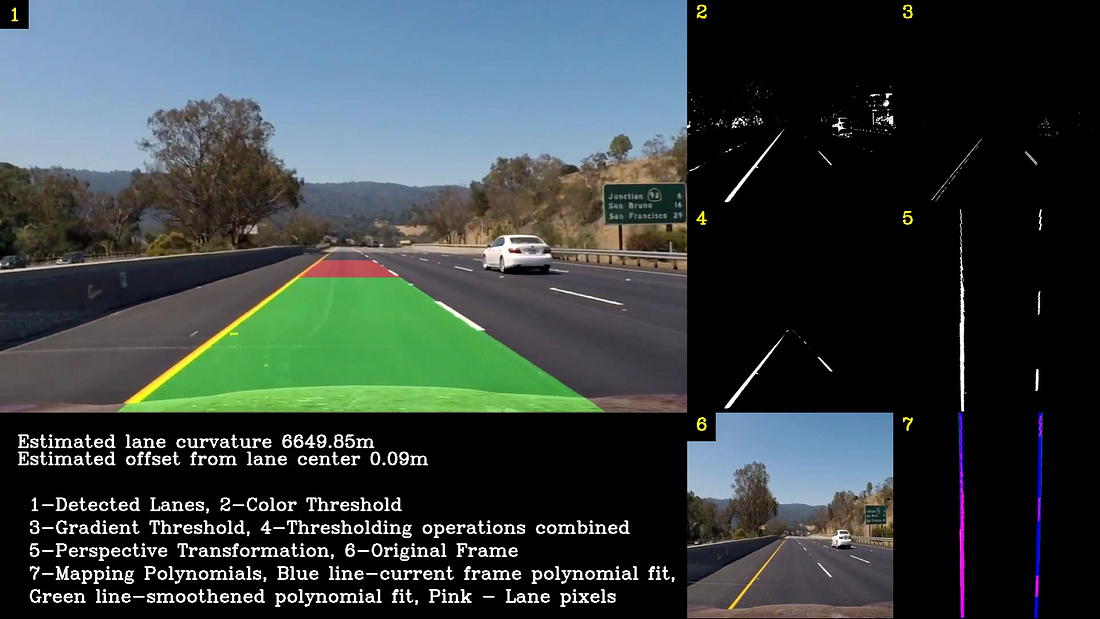The software pipeline consists of the following stages:
1. Compute the camera calibration matrix and distortion coefficients given a set of chessboard images. Apply a distortion correction to raw images.
2. Use color transforms and gradients to create a thresholded binary image.
3. Apply a perspective transform to rectify binary image (“birds-eye view”).
4. Detect lane pixels and fit a polynomial expression to find the lane boundary.
5. Determine the curvature of the lane and vehicle position with respect to center.
6. Overlay the detected lane boundaries back onto the original image.
7. Output visual display of the lane boundaries and numerical estimation of lane curvature and vehicle position in the video.
Let us now discuss each of these Software Pipeline stages in detail.

Step 1: Camera Calibration Stage
Real cameras use curved lenses to form an image, and light rays often bend a little too much or too little at the edges of these lenses. This creates an effect that distorts the edges of images, so that lines or objects appear more or less curved than they actually are. This is called radial distortion, which is the most common type of distortion.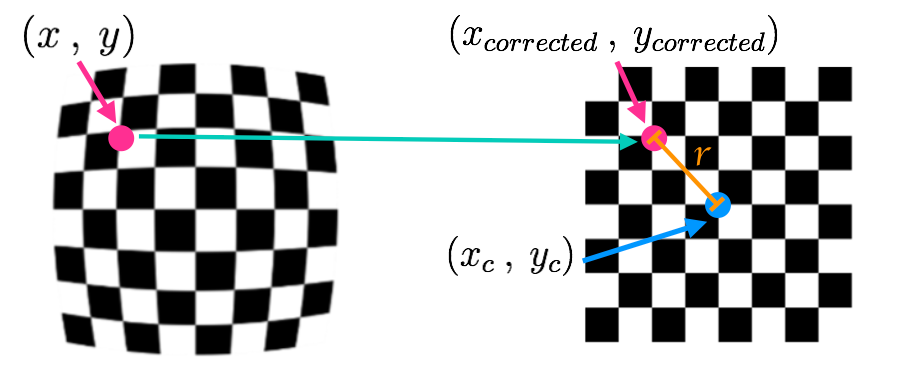Figure 1: Radial distortion correction technique
There are three coefficients needed to correct radial distortion: k1, k2, and k3. To correct the appearance of radially distorted points in an image, one can use a correction formula mentioned in Figure 2.

In the above equations, (x,y) is a point in a distorted image. To undistort these points, OpenCV calculates ‘r’, which is the known distance between a point in an undistorted (corrected) image (xcorrected ,ycorrected) and the center of the image distortion, which is often the center of that image (xc ,yc). This center point (xc ,yc) is sometimes referred to as the distortion center. These points are pictured in Figure 1.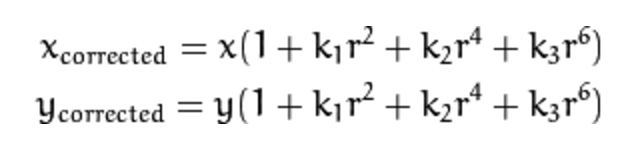Figure 2: Radial Distortion correction formula
In the above equations, (x,y) is a point in a distorted image. To undistort these points, OpenCV calculates ‘r’, which is the known distance between a point in an undistorted (corrected) image (xcorrected ,ycorrected) and the center of the image distortion, which is often the center of that image (xc ,yc). This center point (xc ,yc) is sometimes referred to as the distortion center. These points are pictured in Figure 1.

In the Calibration stage the following operations are performed:

• Finds the chessboard corners.
• Start by preparing object points, which will be the (x, y, z) coordinates of the chessboard corners in the world. Here I am assuming the chessboard is fixed on the (x, y) plane at z=0, such that the object points are the same for each calibration image. Thus, objp is just a replicated array of coordinates, and objpoints will be appended with a copy of it every time I successfully detect all chessboard corners in a test image. imgpoints will be appended with the (x, y) pixel position of each of the corners in the image plane with each successful chessboard detection.
• Performs the cv2.calibrateCamera() to compute the distortion co-efficients and camera matrix that we need to transform the 3d object points to 2d image points.
• Stores the calibration values in a pickle file to use the parameters later.

Step 2: Distortion Correction Stage

Using the distortion co-efficients and camera matrix obtained from the camera calibration stage I undistort the images using the cv2.undistort function. A sample chessboard image and corresponding undistorted image is shown in Figure 3.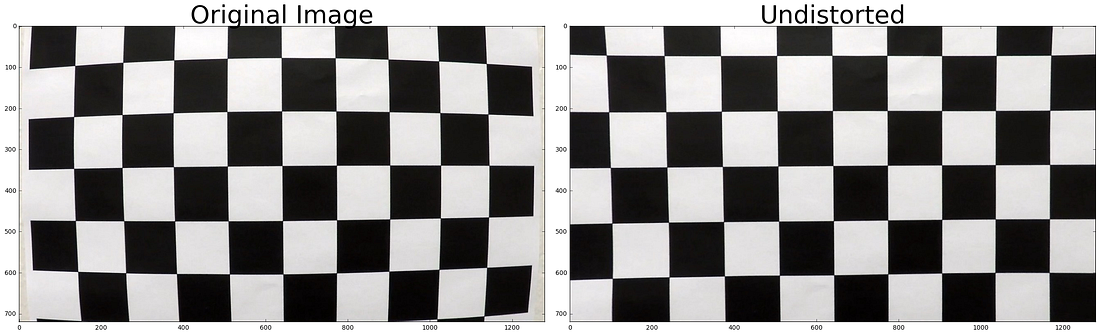Figure 3: Left: Sample chessboard image Right: Undistorted image

By performing the distortion correction we see that the chessboard lines appear to be parallel compared to the original raw image. Another sample image and corresponding undistorted image is shown in Figure 4.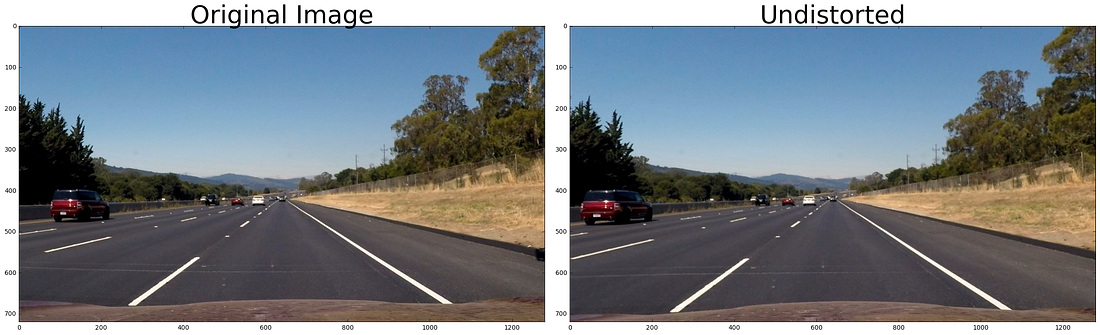Figure 4: Left: Sample car camera image Right: Undistorted image
We see that the car on the left appears to be shifted left compared to the original raw image.

Step 3: Creating a Thresholded binary image using color transforms and gradients

In the thresholding binary image stage, multiple transformations are applied and later combined to get the best binary image for lane detection. Various thresholding operations used are explained below.

Step 3.1: Saturation thresholding:

The images are transformed to HLS color space to obtain the saturation values, the yellow color lanes are best detected in the saturation color space.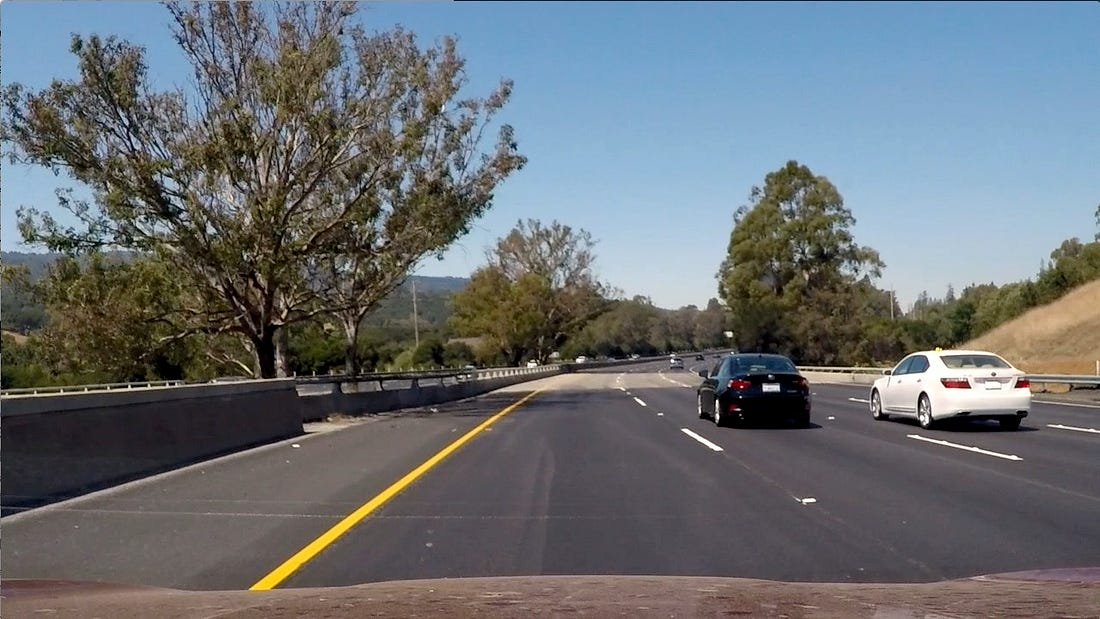Figure 5: Original Image on which Saturation thresholding to be applied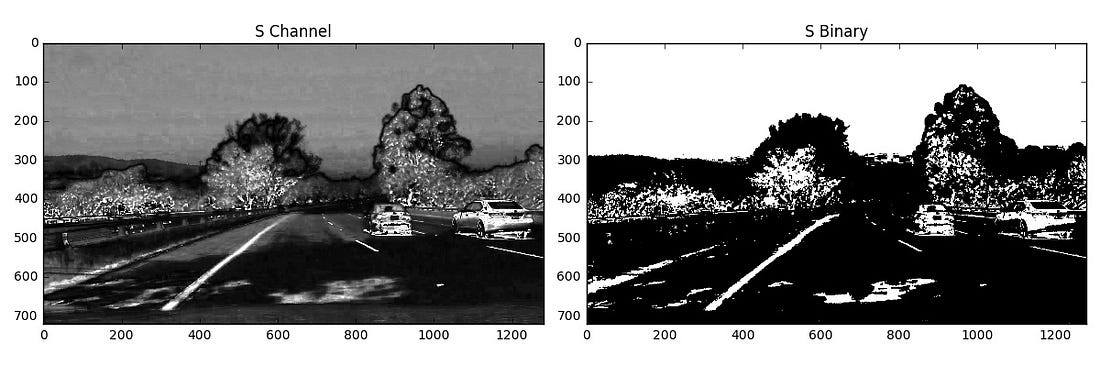Figure 6: Left: Saturation channel of original image Right: Thresholded binary image of the Left image

Step 3.2: Histogram equalized thresholding:

The images are transformed to gray scale and histogram is equalized using the cv2.equalizeHist() function, the white color lanes are best detected using this operation.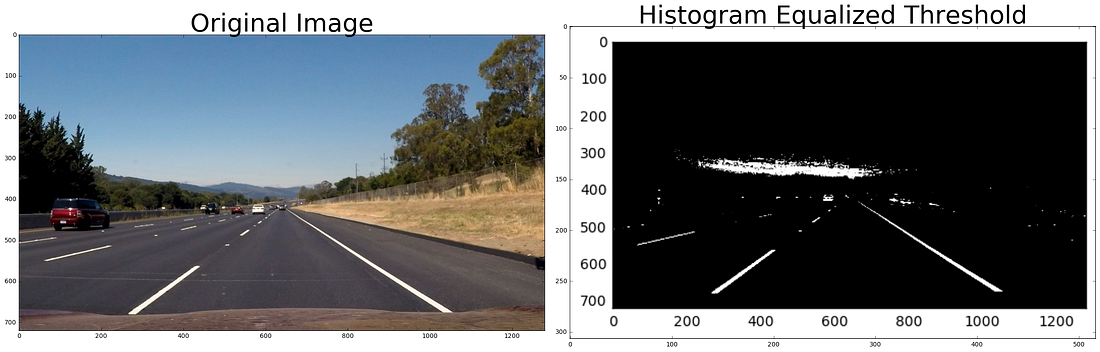Figure 7: Left: Original image Right: Thresholded histogram equalized binary image

The Sobel operator is applied to get the gradients in the x and y direction which are also used to get the magnitude and direction thresholded images. To explain these thresholding I use the below test image and apply the 4 thresholding operations.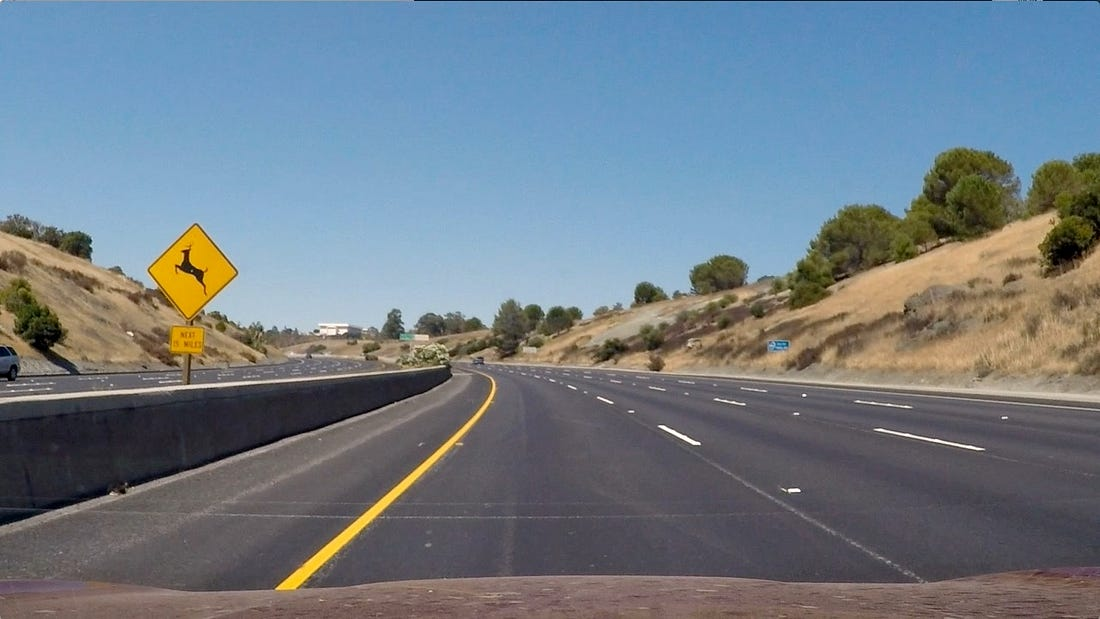Figure 8: Original image on which Gradient thresholding is to be applied
Step 3.3.1: Gradient thresholded in x-direction using Sobel operator.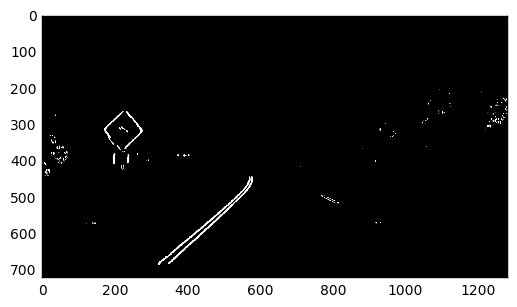Figure 9: Sobel operation on the original image in X-direction to obtain a binary image
Step 3.3.2: Gradient thresholded in y-direction using Sobel operator.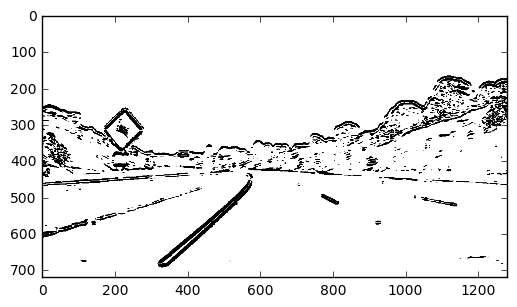Figure 10: Sobel operation on the original image in Y-direction to obtain a binary image
Step 3.3.3: Magnitude threshold of the Sobel Gradient.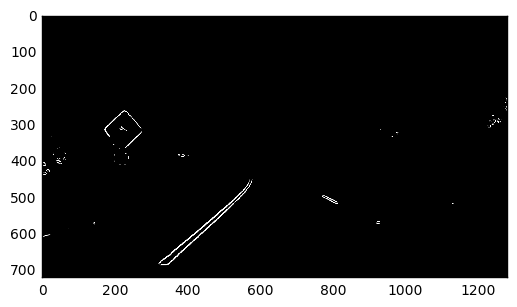Figure 11: Binary thresholded image obtained from the magnitude of the Sobel operation
Step 3.3.4: Direction threshold of the Sobel Gradient.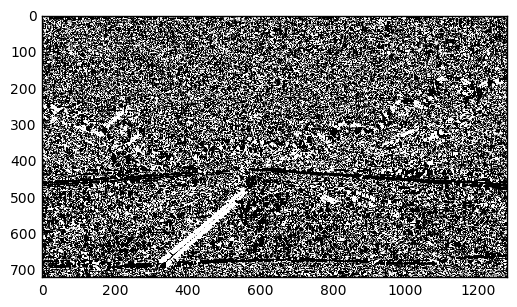Figure 12: Binary thresholded image obtained from the direction value of the Sobel operation

Step 3.4: Region of Interest:

Region of Interest operation is a process of masking unwanted portions of an image, thus keeping only essential part of the image —here the lanes.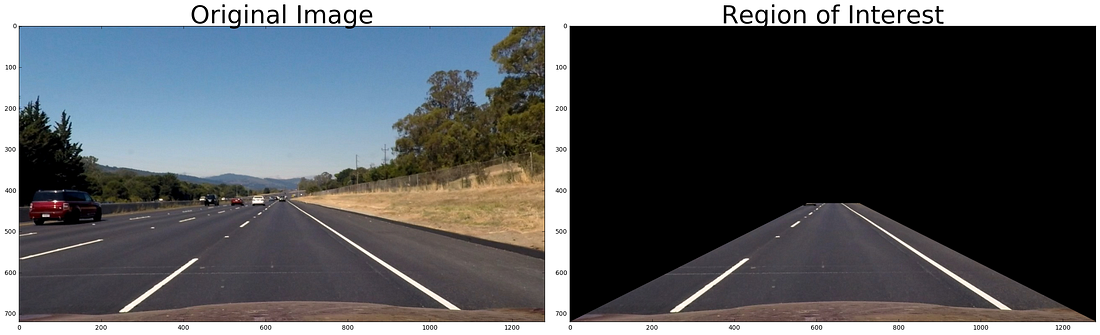The Figure 13 shows the region of interest operation.

To obtain the clear distinctive lanes in the binary image, threshold parameters for the above operation have to be fine tuned. This is the most critical part as the clear visible lanes are easier to detect and fit a polynomial expression in later steps. The fine tuning process is done by interactively varying the threshold values and checking the results as shown below. Here the Region of Interest operation is also implemented to get the best final binary image as shown in the Figure 14.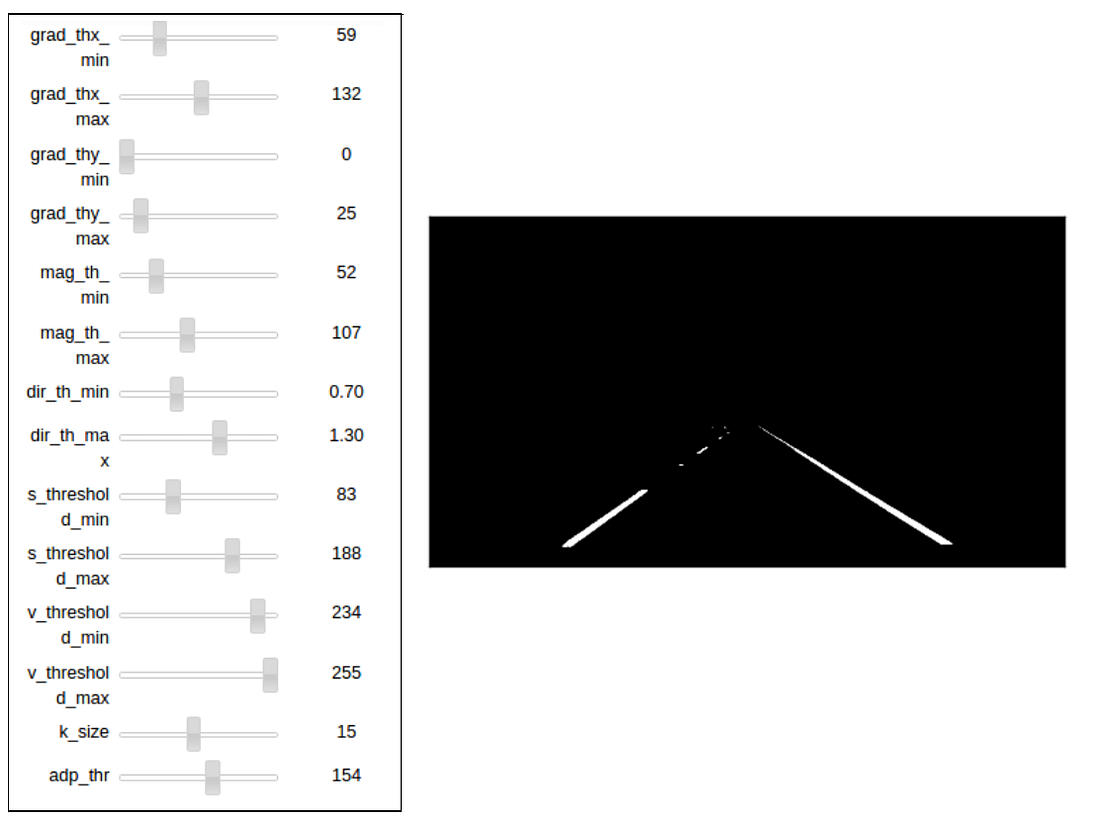Figure 14: Left: Dynamic tool to get the best parameters for the lane detection Right: Best detected lanes after applying the various transformations and thresholding operations.

Step 4: Perspective transformation:

After finalizing the thresholding parameters, we proceed to the next pipeline stage — Perspective transformation.
A perspective transform maps the points in a given image to different, desired, image points with a new perspective. For this project, perspective transformation is applied to get a bird’s-eye view like transform, that let’s us view a lane from above; this will be useful for calculating the lane curvature later on. The source and destination points for the perspective transformation are as shown in the Figure 15.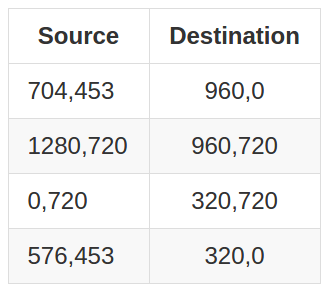Figure 15: The source and destination points for the perspective transformation
The following images shows the perspective transformation from source to destination. Images having parallel lanes as shown in Figure 16 and Figure 17.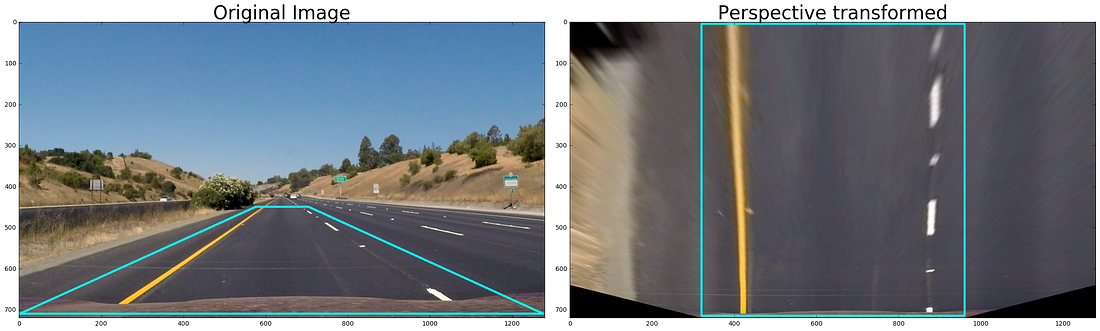Figure 16: Left: Original image with source points marked Right: Perspective transformation on the marked destination points.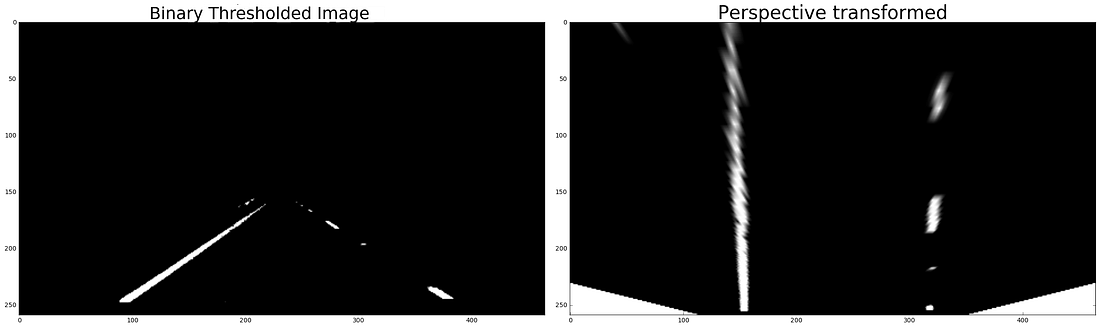Figure 17: Left: Thresholded binary image Right: Perspective transformation destination points.
Image having curved lanes, here lanes appear parallel in normal view(original image), but on perspective transformation we can clearly see that the lanes are curved as shown in Figure 18 and Figure 19.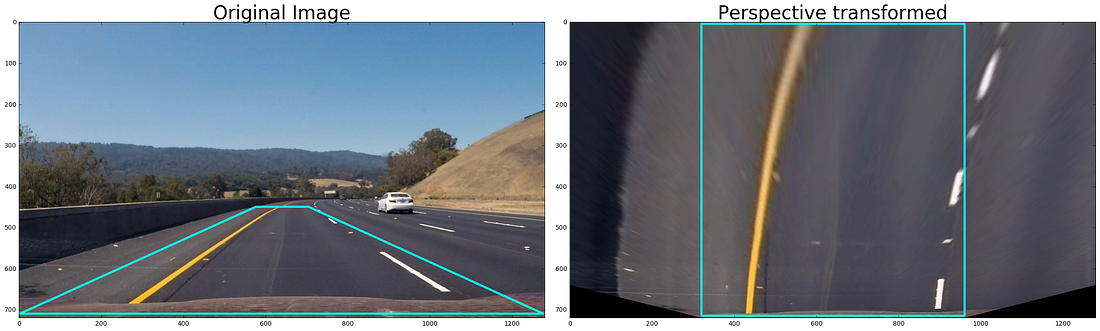Figure 18: Left: Original image with source points marked Right: Perspective transformation on the marked destination points.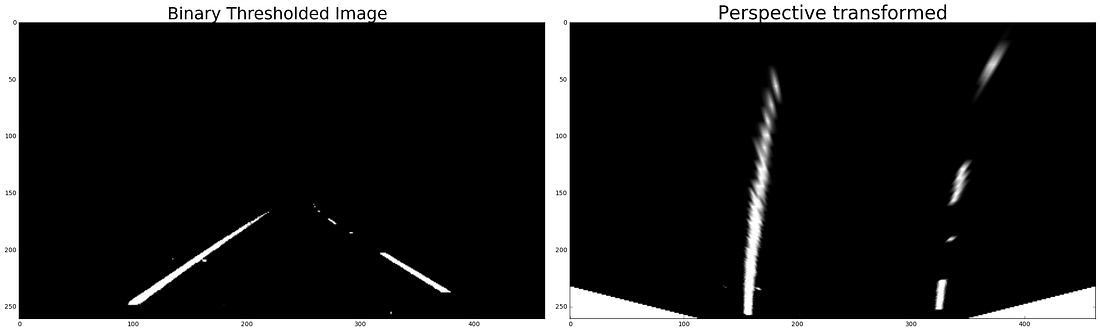Figure 19: Left: Thresholded binary image Right: Perspective transformation destination points.

Step 5: Detect lane pixels and fit to find the lane boundary.

After applying calibration, thresholding, and a perspective transform to a road image, we have a binary image where the lane lines stand out clearly as shown in Figure 19. Next a polynomial curve is fitted to the detected lanes. For this, I first take a histogram along all the columns in the lower half of the image. The histogram plot is shown in Figure 20.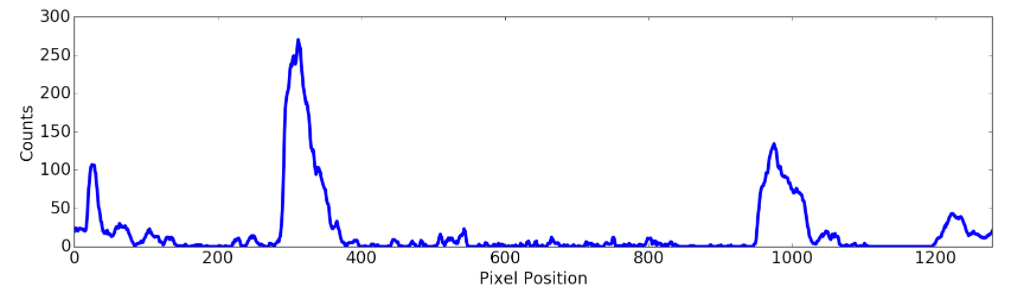Figure 20: Histogram along all the columns in the lower half of the image to find the position of the lanes.
With this histogram, I am adding up the pixel values along each column in the image. In my thresholded binary image, pixels are either 0 or 1, so the two most prominent peaks in this histogram will be good indicators of the x-position of the base of the lane lines. I use that as a starting point to search for the lines. From that point, I use a sliding window, placed around the line centers, to find and follow the lines up to the top of the frame. The sliding window technique can be shown as in the Figure 21.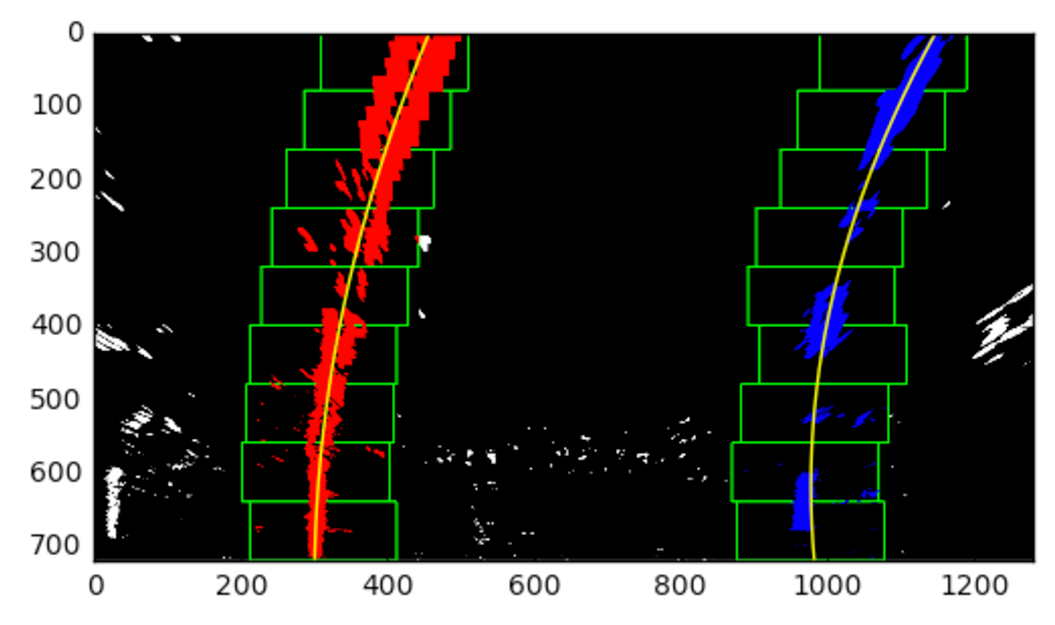Figure 21: Sliding window (in green color), placed around the line centers (yellow lines), to find and follow the lines up to the top of the frame. Red and blue colors represents the lane pixels.
In the Figure 21 the sliding windows are shown in green, left lanes are red colored, right lanes are blue colored and the polynomial fits are yellow lines.

This pipeline when applied to the video frames gives lots of jittering between the frames. To prevent this, I have implemented the smoothing/averaging over 10 previous frames to get a jitter free lane detection. This average valued polynomial fits of previous frames are also used in scenarios where the polynomial fits are not reasonable as shown in the Figure 22.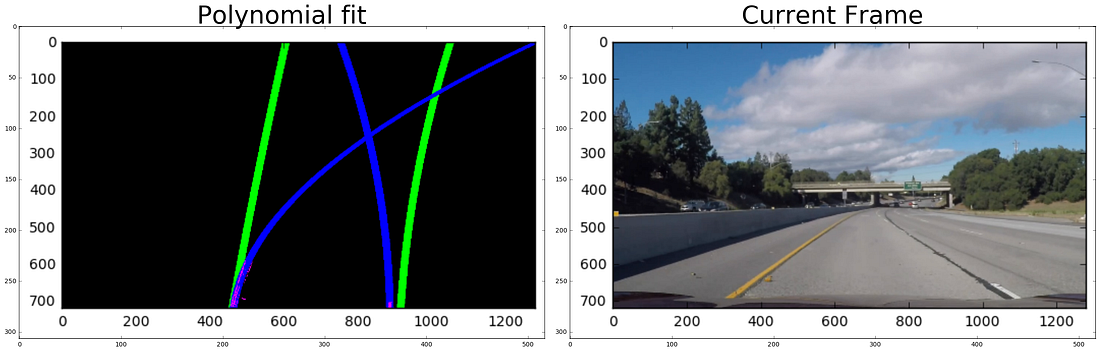Figure 22: Left: Polynomial fits on the lanes detected, blue represents current frame and green for the averaged past frames polynomial fit Right: Current frame of the video
Here the green lines are the polynomial fit of the past 10 frames and the blue represents the polynomial fit for the current frame. The lane pixels are pink colored. It can be observed that the left and right lanes cross each other which is not a practical scenario, so a better judgement call here is to consider the averaged polynomial of the past frames in these cases.

Step 6: Determine the curvature of the lane and vehicle position with respect to center.

The curvature of the lanes f(y) are calculated by using the formula R(curve)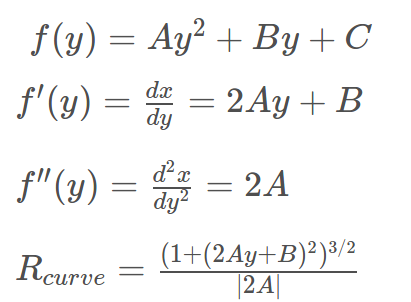Figure 23: Polynomial equation of the lane detected and the radius of curvature
The vehicle position is calculated as the difference between the image center and the lane center.

Step 7: Overlay the detected lane boundaries back onto the original image.

Now we overlay the detected lanes on the original images using inverse perspective transform. The Figure 24 shows the mapping of the polynomial fits on the original image. The region between the lanes are colored green indicating higher confidence region. The region on the farthest end are colored red indicating that the lanes detected are low confidence region.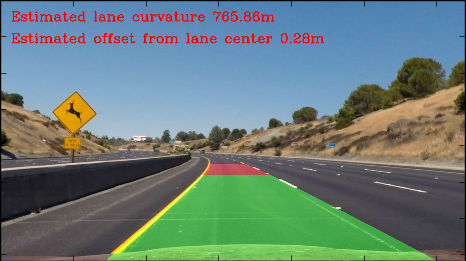Figure 24: Overlay of the lane detected on the original frame along with the values of radius of curvature and offset from center.

Debugging Tools  -  Whats happening behind the scene ??

This project involves fine tuning of lot of parameters like color thresholding, gradient thresholding values to obtain the best lane detection. This can be trickier if the pipeline fails for few video frames. To efficiently debug this I had to build a frame that captures multiple stages of the pipeline, like the color transformation, gradient thresholding, line fitting on present and averaged past frames. The videos of the diagnostic tool are shown below:

Project Video diagnosis:

Challenge Video diagnosis:

Results
The results of the pipeline applied on the project submission video and challenge video are shown below.

Project Video:

Challenge Video:

Key Project Work Learnings and Possible Future Work

• This project involves lot of hyper-parameters that need to be tuned properly to get the correct results. So use of dynamic tools like the one varying hyper parameters to check the output was beneficial.
• The debugging of the project was challenging. Knowing which part of the software pipeline was breaking the code was an essential ingredient for successfully completing the project in time. This was made possible and significantly easier with the help of the diagnosis tool as shown in the diagnosis video.
• The processing of this pipeline on my system is very time consuming, need to check the pipeline on high performance machines with GPU.
• The pipeline fails in low light conditions where the lanes are not visible on lots of continuous frames. This was observed during the testing of the pipeline on low light condition videos.
• This project was based on conventional computer vision techniques, I would like to implement a solution to this problem using techniques from machine learning.
Lane Tracking use OpenCV Python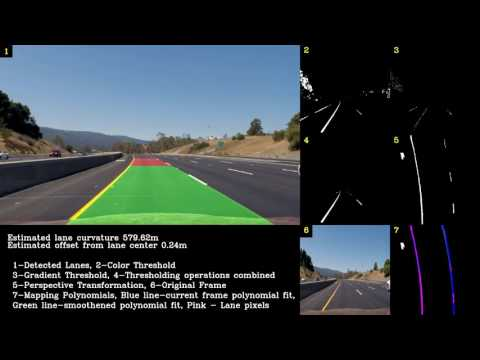Reviewed by Unknown on March 20, 2017 Rating: 5

1.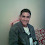hi, can u please help me with my roject using EMGUCV to do something like yours, thank you

1.Hi Vb,
EmguCV seem more difficult to make it happend.Suggest you to use Python or C++